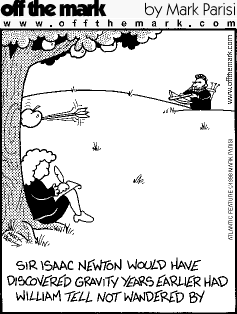This section provides knowledge and understanding of Forces, what a force is and the effects of a force.

Engineers analyse forces when designing a great variety of machines and instruments, from road bridges and fairground rides to atomic force microscopes. Anything mechanical can be analysed in this way. Recent developments in arti cial limbs use the analysis of forces to make movement possible.The different areas of this topic are outlined here;

• Contact and non-contact forces
• Centre of mass (COM)
• Resultant forces
• Resolving forces
• Hooke’s law
• Work done and the definition of the joule
• Pressure
• Pressure at different depths
• How the atmosphere changes at different heights
• Moments and turning forces
• Speed, velocity, distance, displacement and relative speed of objects
• Distance-time graphs
• Velocity-time graphs
• Acceleration
• Equations of motion
• Newtons 1st law
• Newtons 2nd law
• Newtons 3rd law
• Thinking distance, braking distance and stopping distance
• Reaction time, thinking time and braking time
• Momentum
• Conservation of momentum
• Force as a rate of change of momentum

Please remember that you started your GCSEs in year 9 and also learnt material in years 7 and 8 which led into this topic.